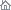Home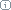About Us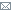ContactBlogWhat's NewProductsBuy NowDownloadsForumGeneXproServer Online Guide Learn how to seamlessly integrate your models with your systems & workflow with the Online Guide.Last update: February 19, 2014GeneXproServer APIIntroduction

With GeneXproServer 5.0 we are introducing an API (Application Programming Interface) that allows you to take control of the process of creating, improving and testing new models as well as scoring data against models and make predictions. You can use the API, for example, when you need to create complex workflows that are not supported by GeneXproServer’s job definition processing.

The focus of this first version of GeneXproServer’s API is simplicity. It defines a small set of operations that can be grasped and put to work in a few minutes if you know how to program in any of the .NET languages such as C#, VB.NET, IronPython or C++ CLI. All code samples in this document are in C#.

Requirements

The GeneXproServer 5.0 API was built against the .NET Framework 4.0. When starting a project you need to add a reference to the library gxps5api.dll that is installed to the folder C:\Program Files (x86)\GeneXproServer 50\ in 64 bits versions of Windows or to C:\Program Files\GeneXproServer 50\ otherwise.

GeneXproServer ships with a sample project with examples of all supported operations that can be found in the folder C:\Program Files (x86)\GeneXproServer 50\samples\GeneXproServerApiSample\ or C:\Program Files\GeneXproServer 50\samples\GeneXproServerApiSample\ assuming you installed GeneXproServer to the default location.

Structure

The current version of the API contains four interfaces and four public classes. The interfaces are IDataset, IRun, IScorer and IPredictor and are implemented internally. The creation of instances that implement these interfaces follows the Factory pattern which is implemented by the RunFactory static class. The classes Model and Statistics are DTOs (Data Transfer Objects) and the RunEventArgs derives from EventArgs and is used to report on the processing engines.

All method calls in the interfaces are blocking calls and the member instances are not thread safe.

Start, Continue, Simplify and Complexify

Opening and starting a run is a very simple operation:

```var run = RunFactory.OpenRun(@"c:\MyRun.gep");
run.Start(50);
Console.WriteLine(run.ActiveModel.TrainingStatistics.Fitness);```

The snippet above opens the run MyRun.gep, processes it for 50 generations and then prints the training fitness to the console.

RunFactory.OpenRun returns an implementation of the interface IRun that allows you to start new runs and continue existing ones, change the current model and test existing models. It also contains a list of all the models in the run and summary information about both the training and validation datasets. Finally, the IRun interface includes an event that you can subscribe to in order to receive notifications of the run processing.

Continuing a run from the active model is also a simple operation:

```var run = RunFactory.OpenRun(@"c:\MyRun.gep");
run.Continue(50);
Console.WriteLine(run.ActiveModel.TrainingStatistics.Fitness);```

This snippet opens the run MyRun.gep and continues improving the active model for 50 generations. It finishes by printing the new training fitness to the console.

Simplify and Complexify operations are similar:

```var run = RunFactory.OpenRun(@"c:\MyRun.gep");
run.Simplify(50);```

and

```var run = RunFactory.OpenRun(@"c:\MyRun.gep");
run.Complexify(50);```

Changing the Active Model

The IRun interface also exposes a way to change the active model:

```var run = RunFactory.OpenRun(@"c:\MyRun.gep");
Console.WriteLine(run.ActiveModel.Index);
run.SelectModel(run.Models);
Console.WriteLine(run.ActiveModel.Index);
```

The code above opens a run, prints the index of the active model, changes the active model to be the first model in the run and then prints the index (which is 1).

Testing a Model

IRun exposes functionality that lets you test a model against the training or validation datasets. This is also a simple operation where you only need to identify the model and the dataset type you want to test:

```var run = RunFactory.OpenRun(@"c:\MyRun.gep");
run.Test(run.Models, DataSetEnum.ValidationSet);
Console.WriteLine(run.Models.ValidationStatistics.Fitness);```

The code above opens the run and tests the model with the Index 4 (note that the Index of a model starts with 1) on the validation set and finally prints the newly evaluated fitness.

Scoring Data

Scoring data is a different operation and it is best done in two phases because initializing the model for scoring is an expensive operation. On the other hand, after initialization is complete, scoring each case is a fast operation. All the initialization process is done by the RunFactory class when you call OpenRunForScoring as seen below:

```var run = RunFactory.OpenRunForScoring (@"c:\MyRun.gep", OutputTypeEnum.RawModel);
var data = new double[] { 5, 1, 1, 1, 2, 1, 3, 1, 1 };
var result = run.Calculate(data);```

The first line opens the run and initializes the active model for scoring and returns an implementation of the IScorer interface. The second line creates some fake data for scoring. Note that the array must have the same number of items as there are variables in the dataset even if they are not being used by that specific model. Finally, scoring a record is just a matter of passing the array to the Calculate method. You can call the Calculate method repeatedly with new records without having to create new instances of IScorer. The IScorer interface has two overloaded Calculate methods. One accepts an array of doubles as in the example above, whereas the second accepts an array of strings. The former should be used when all the variables are numeric and the latter when there are categorical variables or missing values in the dataset. The format of the data must match the format of the training dataset.

Note that the OpenRunForScoring method of the RunFactory class also takes an output type enumeration which matches the "Output Type" in the Model Panel in GeneXproTools. This variable is only important for Logistic Regression and Classification runs. It must be set to RawModel for Regression, Time Series Prediction and Logic Synthesis. The following example has categorical values and the most likely class as the output type:

```var run = RunFactory.OpenRunForScoring (@"c:\MyRun.gep", OutputTypeEnum.MostLikelyClass);
var data = new[] { "b", "30.83", "0", "u", "g", "w", "v", "1.25", "t", "t", "1", "f", "g", "202", "0" };
var result = r.Calculate(data);```

Making Predictions

To make predictions in Time Series Prediction runs you request a different interface (IPredictor) which has a single method called Predict that takes the number of predictions to make and returns an array with the predictions:

```var run = RunFactory.OpenRunForPredictions (@"c:\MyTimSeriesRun.gep");
double[] predictions = run.Predict(5);```

In the example above the returned array contains five predictions.

Model Parameters & Model Statistics

The IRun interface contains a list of models of type List. The Model class contains basic information about the model such as:

• Id (Int32): This is the internal id of the model and it is unique throughout the run.
• Index (Int32): The order number of the model. Corresponds to the model number shown in the History Panel of GeneXproTools and it is also unique.
• Generation (Int32): The generation when the model was created.
• IsActive (Boolean): True if the model is the run’s active model.
• RoundingThreshold (Double): The value of the rounding threshold for Logistic Regression and Classification runs.
• Slope (Double): The value of the slope for Logistic Regression runs.
• Intercept (Double): The value of the intercept for Logistic Regression runs.
• TrainingStatistics (of type Statistics): Summary statistics and performance measures for the training set.
• ValidationStatistics (of type Statistics): Summary statistics and performance measures for the validation set.

The Statistics class has the following members:

• Fitness (double?): The fitness of the model on the dataset; it is null if it has not been calculated yet (validation set only).
• FitnessName (string): The name of the fitness function used to calculate the fitness.
• Accuracy (double?): The accuracy of the model on the dataset; it is null if it has not been calculated yet (validation set only).
• Rsquare (double?): The R-square of the model on the dataset; it is null if it has not been calculated yet (validation set only).
• CorrelationCoefficient(double?): The correlation coefficient of the model on the dataset; it is null if it has not been calculated yet (validation set only).
• TruePositives (int?): The number of true positives (TP) of the model on the dataset; it is null if it has not been calculated yet (validation set only).
• TrueNegatives (int?): The number of true negatives (TN) of the model on the dataset; it is null if it has not been calculated yet (validation set only).
• FalsePositives (int?): The number of false positives (FP) of the model on the dataset; it is null if it has not been calculated yet (validation set only).
• FalseNegatives (int?): The number of false negatives (FN) of the model on the dataset; it is null if it has not been calculated yet (validation set only).
• CalculationErrors (int): The number of calculation errors of the model on the dataset.
• Favorite (double?): The favorite statistic value of the model on the dataset; it is null if it has not been calculated yet.
• FavoriteName (string): The name of the favorite statistic.
• Average (double): The average of the model output on the dataset.
• StandardDeviation (double): The standard deviation of the model output on the dataset.
• Min (double): The minimum value of the model output on the dataset.
• Max (double): The maximum value of the model output on the dataset.

Replacing Dataset & Dataset Information

Each run has at least one dataset (Training) and at most two (Training and Validation). The IRun information contains a Dictionary of these datasets indexed by DataSetEnum. Each dataset is an implementation of the IDataset interface that contains the number of records and variables and the type of the dataset. The interface also allows the replacement of the dataset’s contents with new data from a text file. The new data must have the exact same format but can have any number of records, except zero or 1.

```var run = RunFactory.OpenRun(@"c:\MyRun.gep");
run.Datasets[DataSetEnum.TrainingSet].ReplaceDatasetWith(@"c:\newdata.txt", SeparatorEnum.Tab, true);```

The code above starts by opening the run and then proceeds to replace the training set with data from the text file newdata.txt. The columns in the file are separated by tabs and they have headers since the last argument is true.

See Also:

Related Tutorials:

Related Videos:

 Leave Feedback Please enter the number below using the combo boxes before sending your feedback. 3 8 4 0 1 2 3 4 5 6 7 8 9   0 1 2 3 4 5 6 7 8 9   0 1 2 3 4 5 6 7 8 9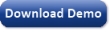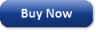Time Limited Trial

Try GeneXproServer for free for 30 days!

Released February 19, 2014

Last update: 5.0.5667GeneXproServerGeneXproToolsSubscribe to the GEP-List
 3 8 4 0 1 2 3 4 5 6 7 8 9   0 1 2 3 4 5 6 7 8 9   0 1 2 3 4 5 6 7 8 9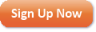Home | What's New | Products | Buy Now | Downloads | Quick Tour | Support | Contact Us | About Gepsoft | Sign UpForum | Blog | Videos | Tutorials | GeneXproTools KB | Logistic Regression Guide | Terms of Use | Privacy & Cookies

Copyright (c) 2000-2014 Gepsoft Ltd. All rights reserved.